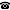# 2019 seminar talk: On convergent sequences of normalised measures on compact spaces

Talk held by Damian Sobota (KGRC) at the KGRC seminar on 2019-06-06.

### Abstract

The celebrated Josefson–Nissenzweig theorem—in a special case of a Banach space $C(K)$ of continuous real-valued functions on an infinite compact Hausdorff space $K$—asserts that there exists a sequence of Radon measures $(\mu_n)$ on $K$ such that the total-variation of each $\mu_n$ is $1$ and for every continuous function $f\in C(K)$ the sequence of the integrals $\int_Kfd\mu_n$ converges to $0$. All the recent natural proofs of the theorem start more or less as follows: "Assume there is not such a sequence $(\mu_n)$ but with an additional property that each $\mu_n$ is a finite linear combination of one-point measures (Dirac's deltas). Then, ..." Although the proofs are correct, it appears that it is not clear at all when this assumption is satisfied. During my talk I will show when (and when not) it is the case that a compact space $K$ admits a such a sequence of measures. As examples Efimov spaces, products of compact spaces, Stone spaces of some funny Boolean algebras will appear.

This is a joint work with Lyubomyr Zdomskyy.

A video recording of this talk is available on YouTube.

Kurt Gödel Research Center for Mathematical Logic. Währinger Straße 25, 1090 Wien, Austria.+43-1-4277-50501. Last updated: 2010-12-16, 04:37.